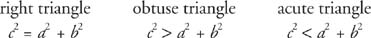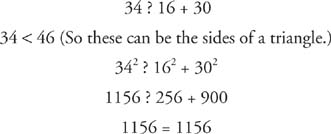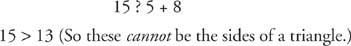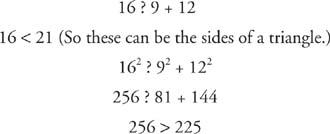## Extension to the Pythagorean Theorem

Variations of Theorem 66 can be used to classify a triangle as right, obtuse, or acute.

Theorem 67: If a, b, and c represent the lengths of the sides of a triangle, and c is the longest length, then the triangle is obtuse if c2 > a2 + b2, and the triangle is acute if c2 < a2 + b2.

Figures 1 (a) through (c) show these different triangle situations and the sentences comparing their sides. In each case, c represents the longest side in the triangle.Figure 1 The relationship of the square of the longest side to the sum of the squares of the other two sides of a right triangle, an obtuse triangle, and an acute triangle.Example 1: Determine whether the following sets of three values could be the lengths of the sides of a triangle. If the values can be the sides of a triangle, then classify the triangle. (a) 16‐30‐34, (b) 5‐5‐8, (c) 5‐8‐15, (d) 4‐4‐5, (e) 9‐12‐16, (f)(Recall the Triangle Inequality Theorem, Theorem 38, which states that the longest side in any triangle must be less than the sum of the two shorter sides.)

a.This is a right triangle. Because its sides are of different lengths, it is also a scalene triangle.

b.This is an obtuse triangle. Because two of its sides are of equal measure, it is also an isosceles triangle.

c.d.This is an acute triangle. Because two of its sides are of equal measure, it is also an isosceles triangle.

e.This is an obtuse triangle. Because all sides are of different lengths, it is also a scalene triangle.

f.This is a right triangle. Because two of its sides are of equal measure, it is also an isosceles triangle.